# Cupid’s Carpet Fractal

#### Project Idea

I call this carpet fractal “Cupid’s” because the holes in the center and the overall outline of the carpet resemble hearts.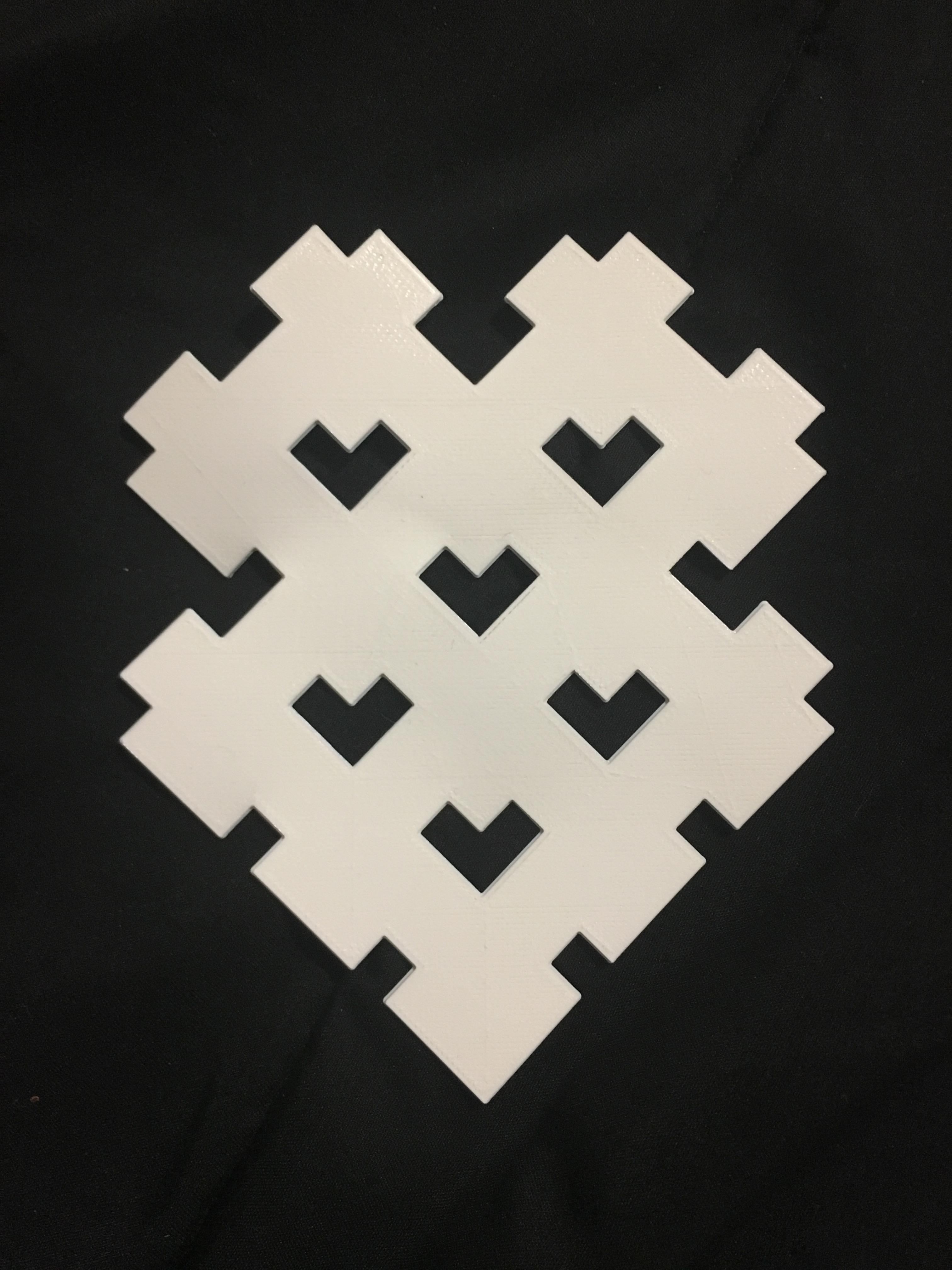I came up with the design simply by playing around with the template cells. I created this shape by indenting [0,0], [3,0] and [0,3]. This is a level 2 print.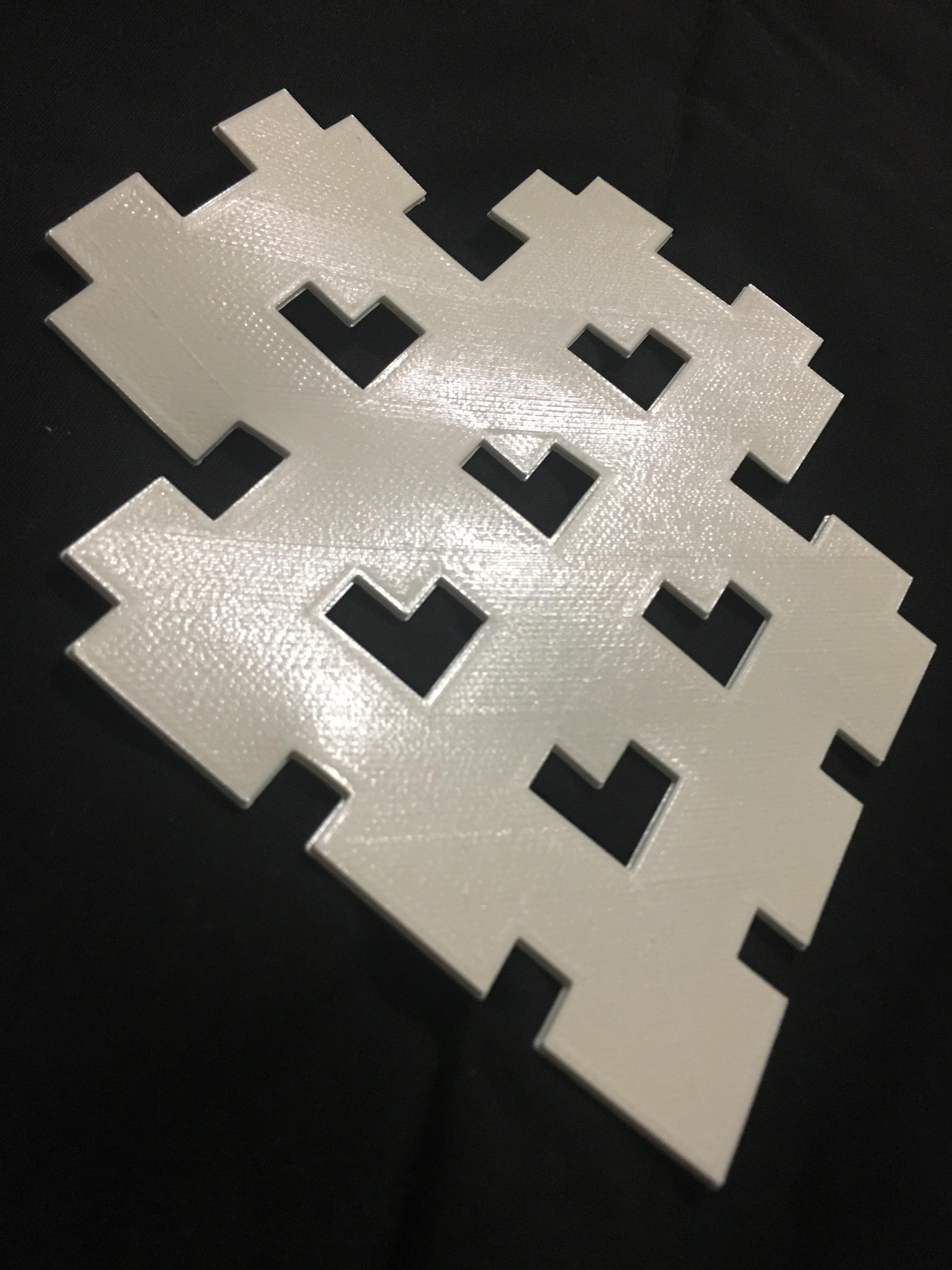#### Design

Level 1: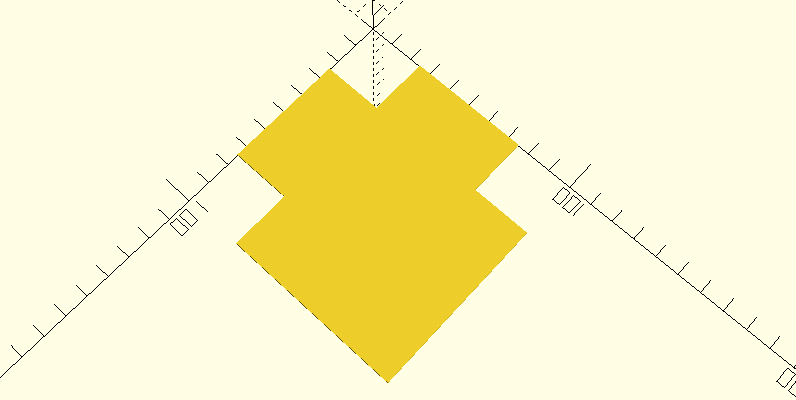Level 2: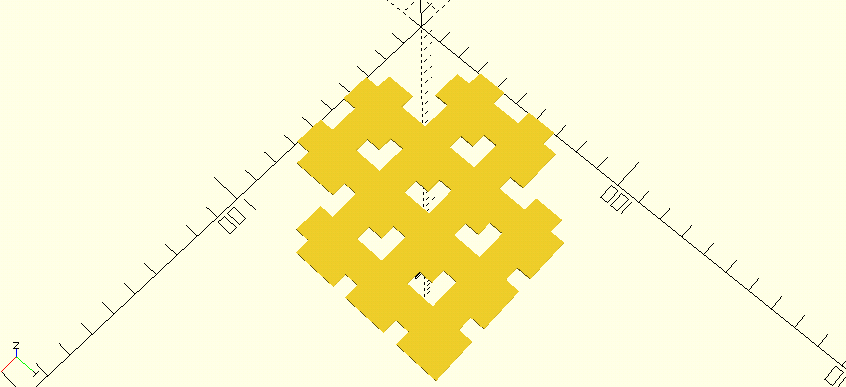Level 3: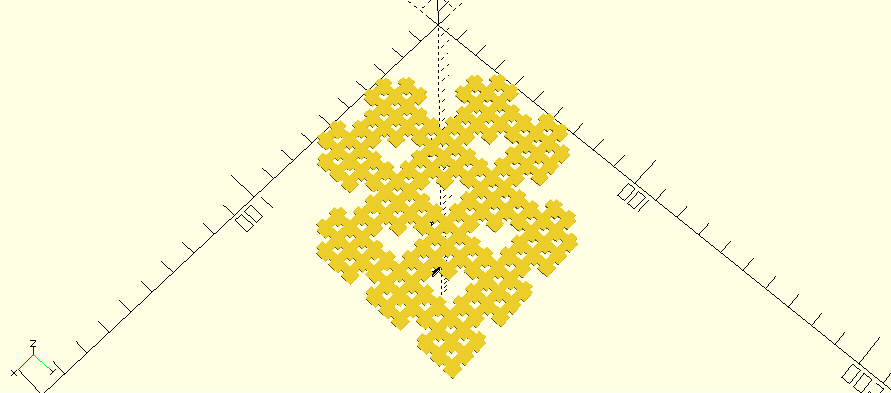#### Math

To calculate the surface area for each of these levels, I began with level 2. I concluded a solid square the size of this fractal is made up of 16 square boxes, which are each made up of 16 smaller square boxes. Because there are holes the size of the smaller boxes in this level, I used that size as the universal unit for all levels’ calculations.

I calculated how many small boxes were in the solid square: 256. I then deducted the number of missing small boxes from that square. Then I took out the three large boxes, dividing each of them into 16 small boxes and subtracted that from the total. The end result is that the surface area of the second level is 169 units (small boxes).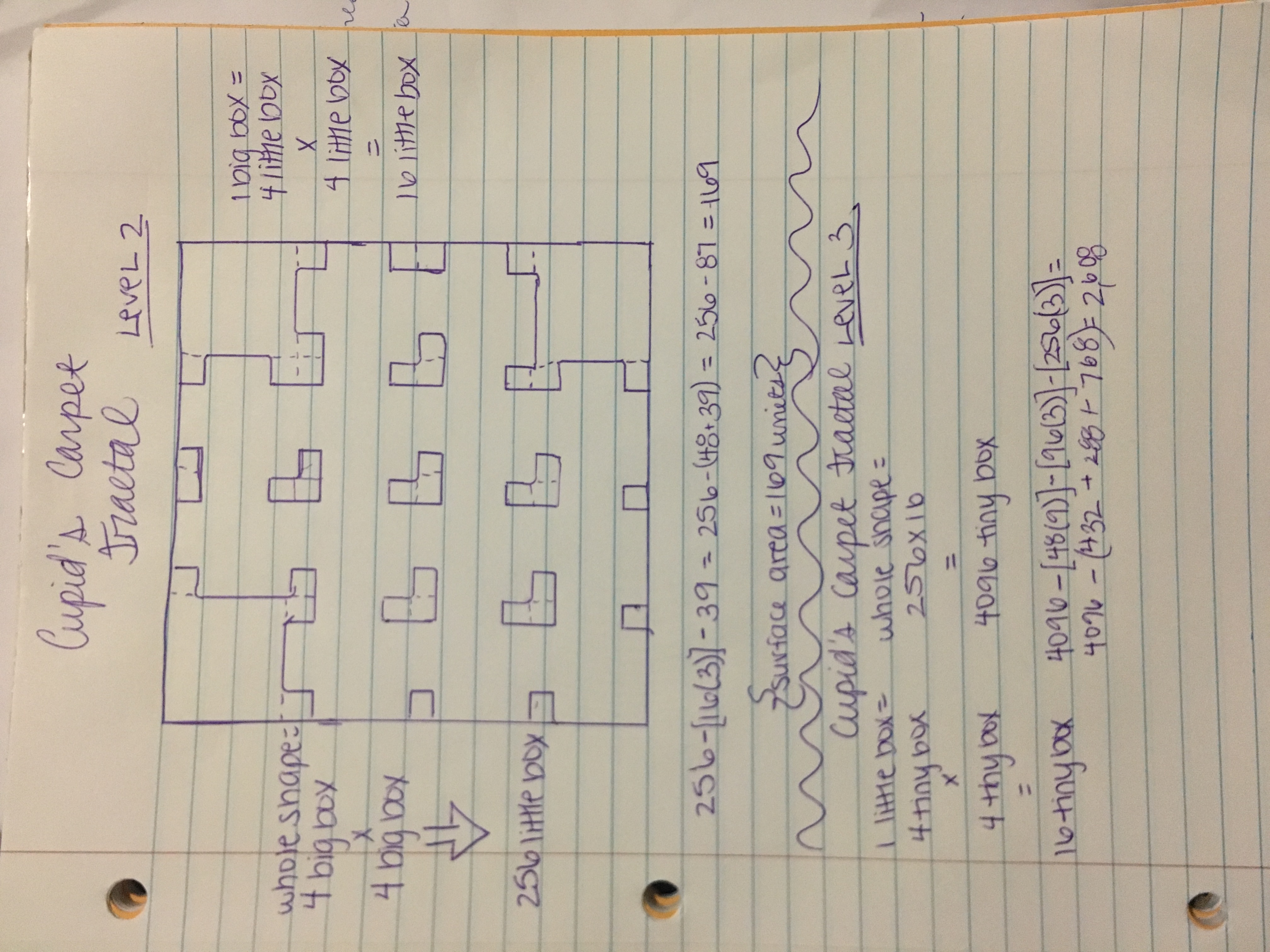##### Equation (Level 2):

256 – [16(3)] – 39

256 – (48 + 39)

256 – 87 = 169

For level 1, I used the same theory that each large box is equal to 16 smaller boxes. To keep units the same in each level, I subtracted 48 from 256. Again, 256 is the total number of small boxes in the full solid square, and there are 16 small boxes in each of  the 3 large boxes (16×3). The surface area came out to 208 units (small boxes)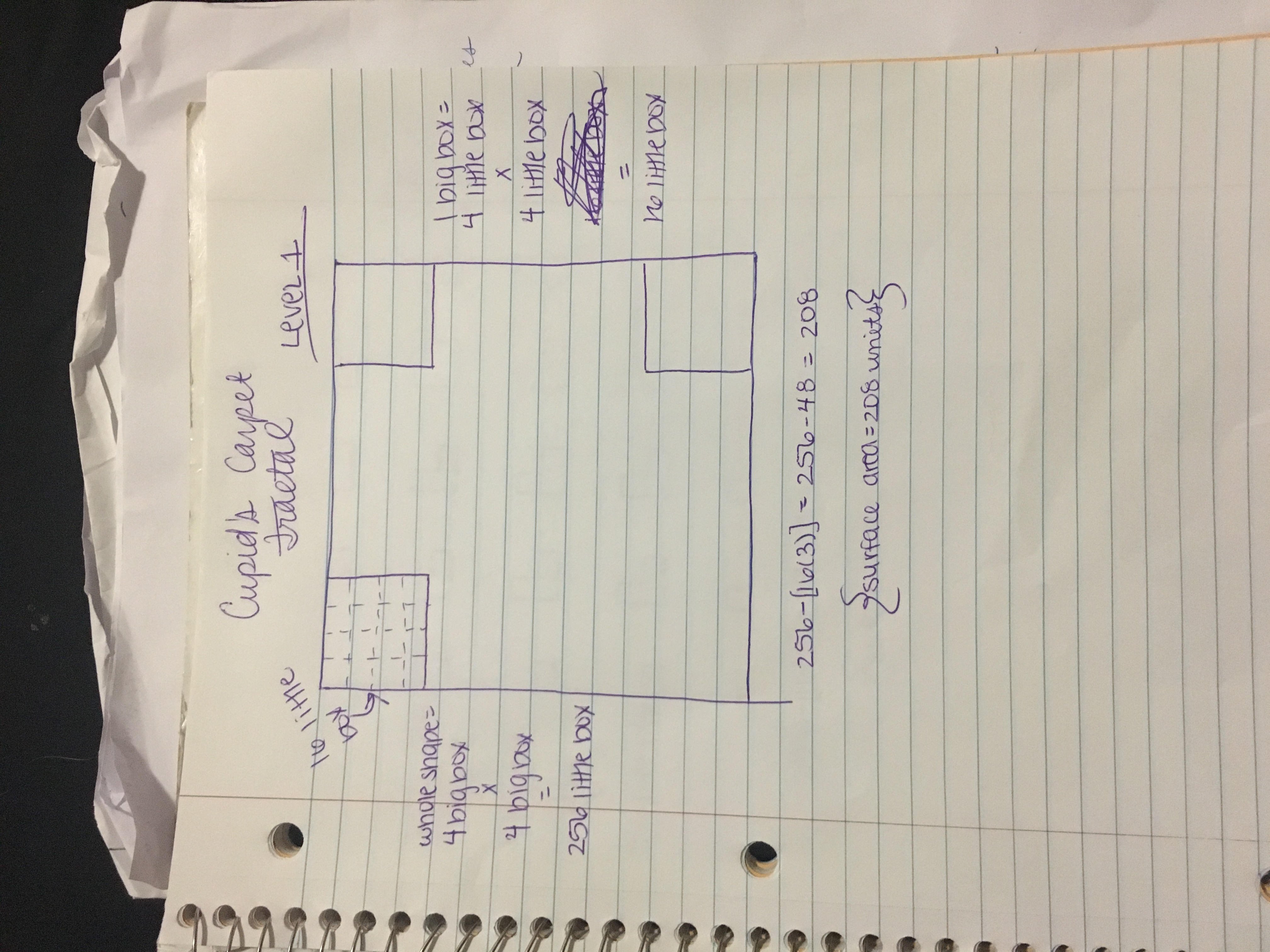##### Equation (Level 1):

256 – [16(3)]

256 – 48 = 208

Finally, to calculate the surface area of level 3, I added in a third level of measurement: tiny boxes. Because I do not yet have a printed copy of this level, the math was very difficult; there are so many tiny boxes, which contributed to my own mechanical error. I did, however, create an equation to start off the search for the surface area for level 3. I

I calculated that the entire solid square was made up of 16 large boxes, 256 small boxes and 4,096 tiny boxes. I broke up each section into tiny boxes to calculate how many were being subtracted from the whole square with each hole. So far the surface area is 2608 tiny boxes or 163 units (small boxes).

##### Equation (Level 3):

4096 – [48(9)] – [96(3)] – [256(3)]

4096 – (432 + 288 + 768)

4096 – 1488 = 2608